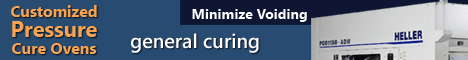Printed Circuit Board Assembly & PCB Design Forum

SMT electronics assembly manufacturing forum.

# Calcutating Surface Tension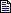How can you determine if a SMT component will have enough s... - Oct 26, 2000 by lefebvres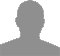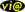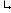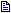Hello: I have not seen a published formula for calculating... - Oct 26, 2000 by ptvianclefebvres

Calcutating Surface Tension | 26 October, 2000

How can you determine if a SMT component will have enough surface tension to stay on the PCB during reflow process? I understand there is a formula that will provide this information.

ptvianc

Re: Calcutating Surface Tension | 26 October, 2000

Hello:

I have not seen a published formula for calculating the package weight that can be secured by molten solder. I have seen forumlas printed for tombstoning. From that aspect, we developed the following rule-of-thumb for first-order estimates:

m = [0.87 gamma(LF) P N]/980

where m = mass (grams) 0.87 is a "safety factor" that assumes a maximum contact angle (theta) of 30 deg so that 0.87 = cos (30 deg). gamma(LF) is the solder surface tension WITH THE FLUX; for Sn-Pb, thats about 380 dynes/cm with an RMA flux P is the wetted perimeter of the I/O (cm) N is the number of wetted I/Os. 980 is acceleration due to gravity (980 cm/s^2).

Give it a try.

Thanks.